NEET  >  Types & Nature of Waves

# Types & Nature of Waves - Notes | Study Physics Class 11 - NEET

 Table of contentsWaveNature of WavesSome Important Terms of Wave MotionSound WavesVelocity of Longitudinal (Sound) Waves1 Crore+ students have signed up on EduRev. Have you?

Wave

A wave is a vibratory disturbance in a medium which carries energy from one point to another point without any actual movement of the medium. There are three types of waves:

• Mechanical Waves: Those waves which require a material medium for their propagation are called mechanical waves, e.g., sound waves, water waves etc.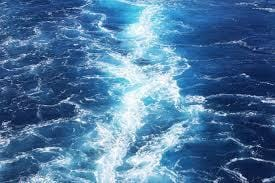Water Waves
• Electromagnetic Waves: Those waves which do not require a material medium for their propagation are called electromagnetic waves, e.g., light waves, radio waves etc.
• Matter Waves: These waves are commonly used in modern technology but they are unfamiliar to us. These waves are associated with electrons, protons and other fundamental particles.

Nature of Waves

Transverse Waves

A wave in which the particles of the medium vibrate at right angles to the direction of propagation of wave is called a transverse wave.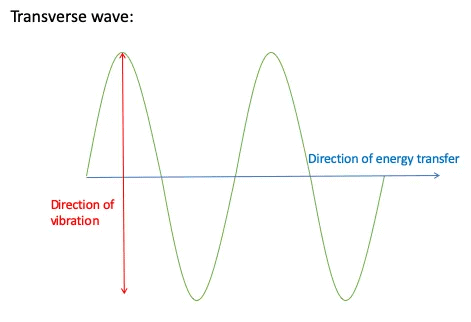These waves travel in the form of crests and troughs.

Longitudinal Waves

A wave in which the particles of the medium vibrate in the same direction in which the wave is propagating is called a longitudinal wave.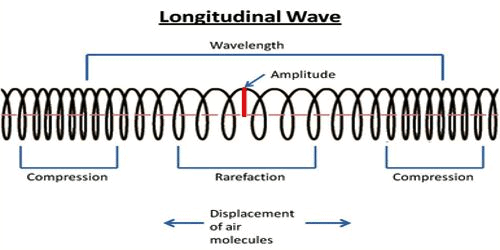These waves travel in the form of compressions and rarefactions.

Some Important Terms of Wave Motion

1. Wavelength: The distance between two nearest points in a wave which are in the same phase of vibration is called the wavelength (λ).
2. Time Period: Time taken to complete one vibration is called the time period (T).
3. Frequency: The number of vibrations completed in one second is called the frequency of the wave.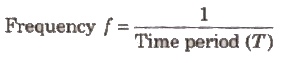Its SI unit is hertz.
4. The velocity of Wave or Wave Velocity: The distance travelled by a wave in one second is called the velocity of the wave (u).
Relation among velocity, frequency and wavelength of a wave is given by v = fλ.
5. Particle Velocity The velocity of the particles executing SHM is called particle velocity.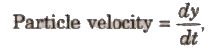Sound Waves

Sound waves of all the mechanical waves that occur in nature, the most important in our everyday lives are longitudinal waves in a medium, usually, air, called sound waves.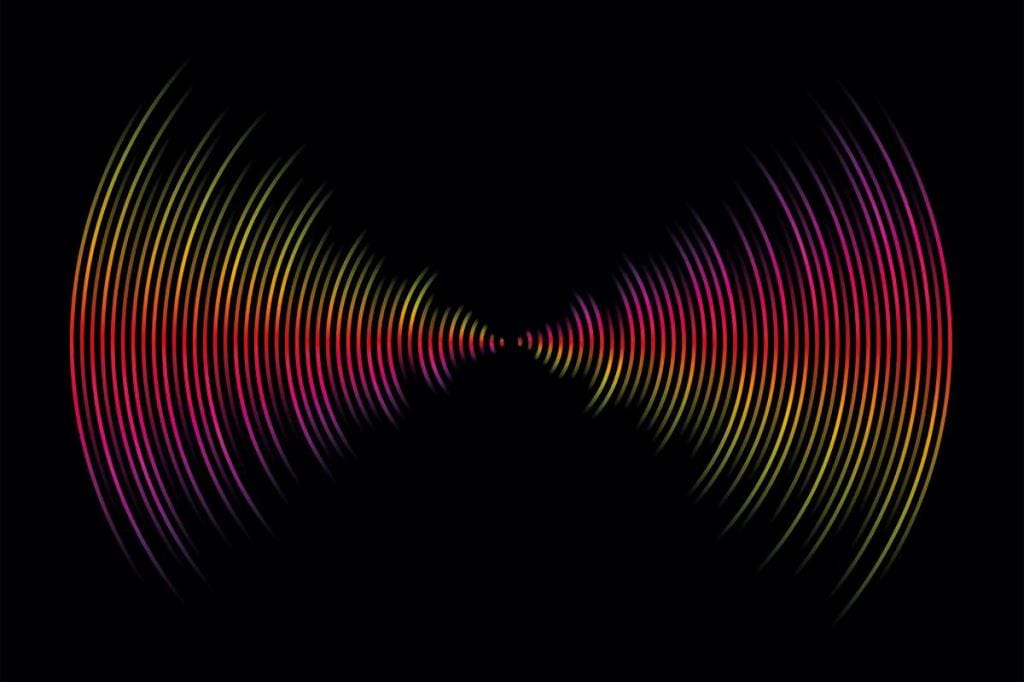Sound waves

Sound Waves are of three types

• Infrasonic Waves: The sound waves of frequency lies between 0 to 20 Hz are called infrasonic waves.
• Audible Waves: The sound waves of frequency lies between 20 Hz to 20000 Hz are called audible waves.
• Ultrasonic Waves: The sound waves of frequency greater than 20000 Hz are called ultrasonic waves.

Sound waves are mechanical longitudinal waves and require medium for their propagation.

[sound waves cannot propagate through vacuum.

If Vs, Vi and Vg are speed of sound waves in solid, liquid and gases, then

Vs > Vi > Vg

Sound waves (longitudinal waves) can reflect, refract, interfere and diffract but cannot be polarised as only transverse waves can polarised.

Velocity of Longitudinal (Sound) Waves

Velocity of longitudinal (sound) wave in any medium is given by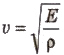where E is the coefficient of elasticity of the medium and ρ is density of the medium.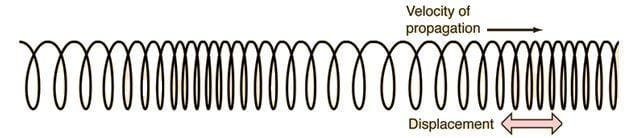Longitudinal waves

Newton’s Formula

According to Newton, the propagation of longitudinal waves in a gas is an isothermal process. Therefore, velocity of longitudinal (sound) waves in gas should be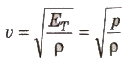where ET is the isothermal coefficient of volume elasticity and it is equal to the pressure of the gas.

Laplace’s Correction

According to Laplace, the propagation of longitudinal wave is an adiabatic process. Therefore, velocity of longitudinal (sound) wave in gas should be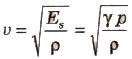where, ES, is the adiabatic coefficient of volume elasticity and it is equal to γ p.

Factors Affecting Velocity of Longitudinal (Sound) Wave

(i) Effect of Pressure: The formula for velocity of sound in a gas.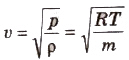Therefore, (p/ρ) remains constant at a constant temperature.

Hence, there is no effect of pressure on velocity of longitudinal wave.

(ii) Effect of Temperature: Velocity of longitudinal wave in a gas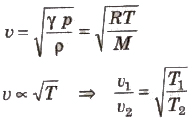Velocity of sound in a gas is directly proportional to the square root of its absolute temperature.

If v0 and vt are velocities of sound in air at O°C and t°C, then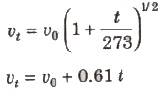(iii) Effect of Density: The velocity of sound in gaseous medium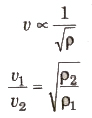The velocity of sound in a gas is inversely proportional to the square root of density of the gas.

(iv) Effect of Humidity: The velocity of sound increases with increase in humidity in air.

Shock Waves

If speed of a body in air is greater than the speed of sound, then it .s called supersonic speed. Such a body leaves behind it a conical region of disturbance which spreads continuously. Such a disturbance is called a shock wave.

The document Types & Nature of Waves - Notes | Study Physics Class 11 - NEET is a part of the NEET Course Physics Class 11.
All you need of NEET at this link: NEET

## Physics Class 11

127 videos|464 docs|210 tests
 Use Code STAYHOME200 and get INR 200 additional OFF

## Physics Class 11

127 videos|464 docs|210 tests

### How to Prepare for NEET

Read our guide to prepare for NEET which is created by Toppers & the best Teachers

Track your progress, build streaks, highlight & save important lessons and more!

,

,

,

,

,

,

,

,

,

,

,

,

,

,

,

,

,

,

,

,

,

;